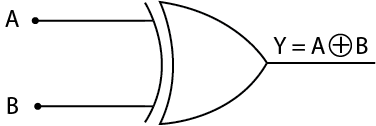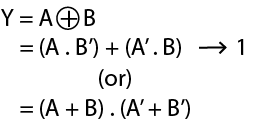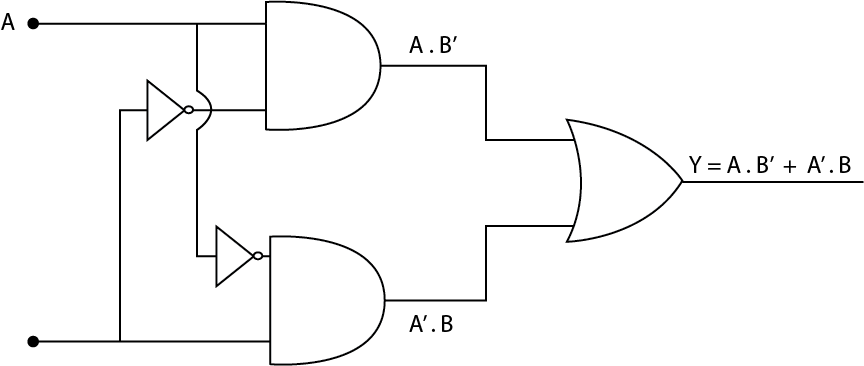Related Tags

python
community creator

# How to implement XOR gate in Python

## What is an Exclusive OR Gate?

The Exclusive-OR Gate/ XOR Gate is a combination of all the three basic gates (NOT, AND, OR gates). It receives two or more input signals but produces only one output signal.

It results in a low '0' if the input bit pattern contains an even number of high '1' signals.

If there are an odd number of high '1' signals, it results in high. For this reason, the Exclusive-OR Gate(XOR Gate) is called the Anti-Coincidence Gate or Inequality Detector or Odd Ones Detector.

Exclusive-OR SymbolInternal CircuitImplementing XOR Logic Gate

From Equation (A . B’) + (A’. B)Truth Table for XOR Gate

A B Y = A.B’ + A’.B
0 0 0
0 1 1
1 0 1
1 1 0

From the Truth Table above, we can observe that the XOR gate gives a high output when there is an odd number of 1's.

## Code

def XOR (a, b):
if a != b:
return 1
else:
return 0
print("|-------------------------|")
print("|        XOR GATE         |")
print("|-------------------------|")
print("|   A   |   B   |    X    |")
print("|_________________________|")
print("|   0   |   0   |","  ",XOR(0,0) ,"   |")
print("|_________________________|")
print("|   0   |   1   |","  ",XOR(0,1) ,"   |")
print("|_________________________|")
print("|   1   |   0   |","  ",XOR(1,0) ,"   |")
print("|_________________________|")
print("|   1   |   1   |","  ",XOR(1,1) ,"   |")
print("|_________________________|")
Output for a 2-input XOR Gate

RELATED TAGS

python
community creator

CONTRIBUTOR